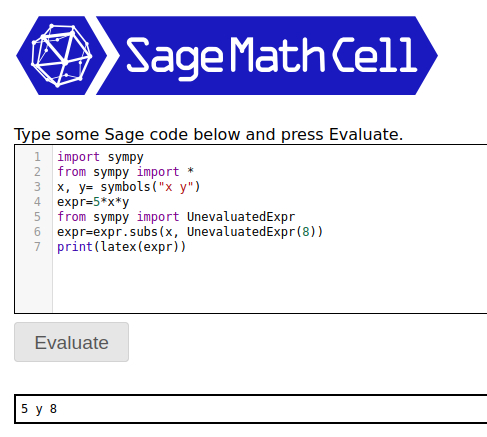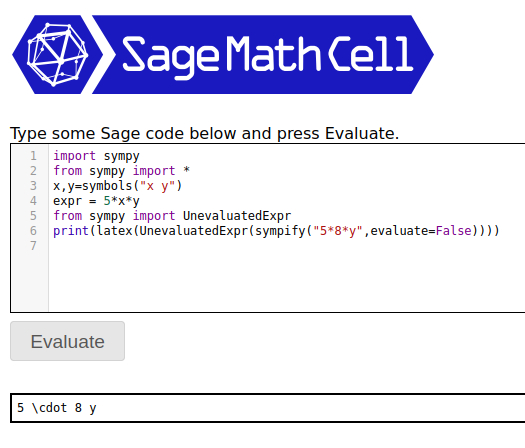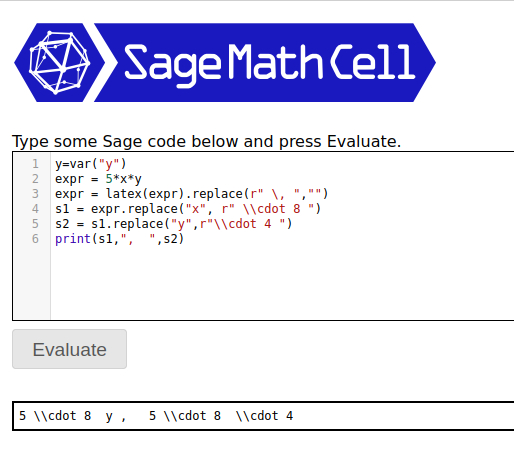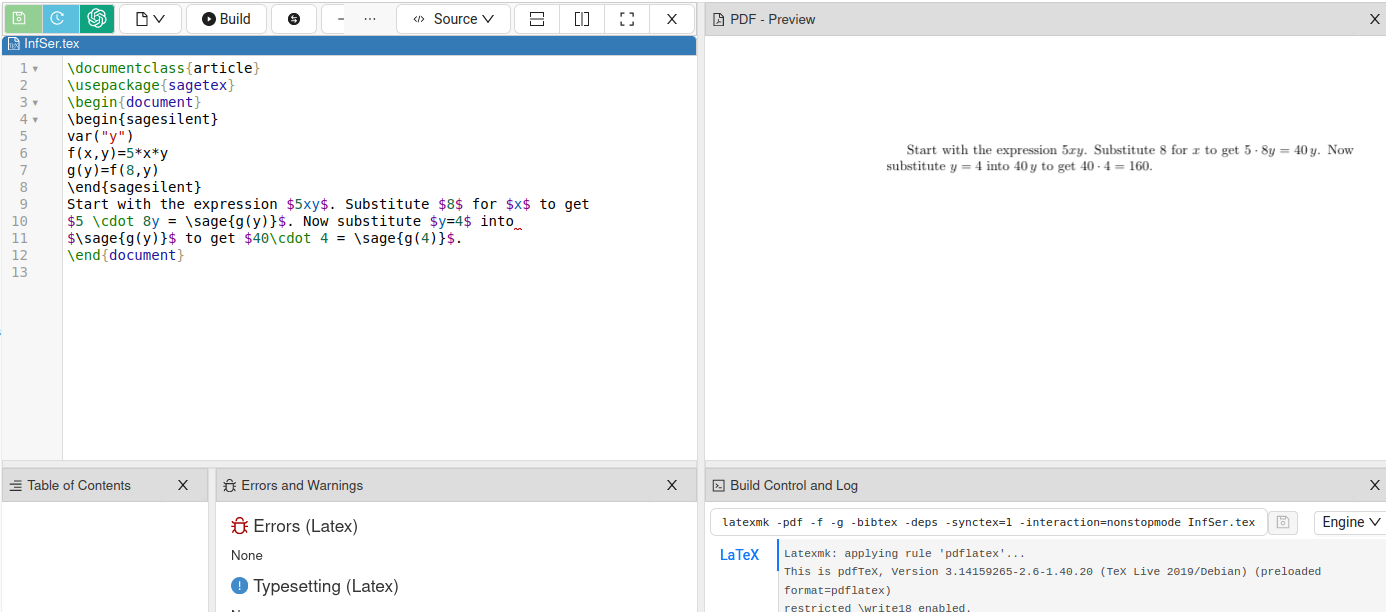# How to substitute a value into an expression without evaluation to convert it to LaTex?

How can I convert an expression to LaTex while substituting a variable value without evaluating the expression after substitution?

For example, I have an expression 5*x*y. The latex(5*x*y) is 5 \, x y as expected. But now I want to substitute a constant instead of a variable. For example 8 instead of x. The LaTex representation (latex((5*x*y).subs(x=8))) of which is would be 40 \, y, so the expression is automatically evaluated. But I want to get something like 5 \\cdot 8 y instead. Is that possible to do with SageMath?

I know something like that exists in SymPy. For example, take a look at the answer to:

It uses sympy.UnevaluatedExpr to wrap an expression to substitute the variable with. Is there anything like that in SageMath?

edit retag close merge delete

Sort by » oldest newest most voted

There are several ways to do it and you'll have to decide on which suits you best. First method: Direct use of sympy. This didn't work out the way I expected: it switched x and y after substitution:If that's okay then maybe this is good enough. I wouldn't want it swapping terms, so with a bit of fiddling came up with this, which is getting more involved:

import sympy
from sympy import *
x,y=symbols("x y")
expr = 5*x*y
from sympy import UnevaluatedExpr
print(latex(UnevaluatedExpr(sympify("5*8*y",evaluate=False))))


Now the order is correct but latex(UnevaluatedExpr(sympify("5*8*y",evaluate=False))) is a bit annoyingNote removing print from the last statement gives you your double escape: '5 \\cdot 8 y'

Second method is to work in strings. This will give you complete control over your output:

y=var("y")
expr = 5*x*y
expr = latex(expr).replace(r" \, ","")
s1 = expr.replace("x", r" \\cdot 8 ")
s2 = s1.replace("y",r"\\cdot 4 ")
print(s1,",  ",s2)


The output is:s1 is the output after the first step of substituting x for r" \cdot 8 " while s2 would be a potential second step of substituting y for r"\cdot 4 ".

Third method, which might be useful depending on the context of how you are using the output, is to work directly in LaTeX and borrow from Sage as needed. This is typically how I work with both. Here is a sample LaTeX document:

\documentclass{article}
\usepackage{sagetex}
\begin{document}
\begin{sagesilent}
var("y")
f(x,y)=5*x*y
g(y)=f(8,y)
\end{sagesilent}
Start with the expression $5xy$. Substitute $8$ for $x$ to get
$5 \cdot 8y = \sage{g(y)}$. Now substitute $y=4$ into
$\sage{g(y)}$ to get $40\cdot 4 = \sage{g(4)}$.
\end{document}more

I found another solution, which is better for me. It still requires me to use sympy, which is unfortunate because sympy symbols are incompatible with sage function declarations (TypeError: must construct a function with symbolic variables as arguments), but it's better than nothing.

There is a global thread-local parameters object sympy.core.parameters.global_parameters with a evaluate parameter that controls whether expressions should be evaluated or not. We can explicitly change the value of evaluate parameter:

sympy.core.parameters.global_parameters.evaluate = False


Or do the same, but using context manager class sympy.evaluate that pushes the evaluate parameter value for us:

with sympy.evaluate(False):
print(x + x)


The evaluate global parameter is restored to the previous value once the code leaves the with statement.

This method is better than using UnevaluatedExpr because it doesn't mess with the order of expressions. For instance:

sympy.latex((5*x*y).subs(x, sympy.UnevaluatedExpr(8)))
'5 y 8'


The order of x (substituted with 8) and y has been changed.

with sympy.evaluate(False):
print(sympy.latex((5*x*y).subs(x, 8)))

5 \cdot 8 y


The order has not been changed.

I could not find such global parameter in sage. hold argument is False by default (from sage source code):

if kwds.get('hold', False):


While in sympy the default value of hold's alternative evaluate argument is the global parameter evaluate:

eval = options.pop('evaluate', global_parameters.evaluate)


I wish I was wrong and there was actually any way to globally disable expressions evaluation in sagemath. I'm still looking for it :)

more

Sage has a "hold" context for some operations, but I don't see how to use it to answer the question.

Especially since the hold context seems quite fragile with respect to substitution.

As a workaround, one can use SymPy inside Sage.

Here are some things one can do with "hold", in case it helps.

sage: a = x
sage: a = a.mul(8, hold=True)
sage: a = a.mul(5, hold=True)
sage: a
5*(8*x)
sage: a.unhold()
40*x

more